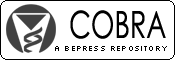## COBRA Preprint Series

#### Abstract

We present a new method for constructing exact distribution-free tests (and con…fidence intervals) for variables that can generate more than two possible outcomes. This method separates the search for an exact test from the goal to create a non- randomized test. Randomization is used to extend any exact test relating to means of variables with fi…nitely many outcomes to variables with outcomes belonging to a given bounded set. Tests in terms of variance and covariance are reduced to tests relating to means. Randomness is then eliminated in a separate step. This method is used to create con…fidence intervals for the difference between two means (or variances) and tests of stochastic inequality and correlation.

#### Disciplines

Statistical Methodology | Statistical Theory

COinS